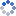加载中…MATLAB技术论坛
• 博客等级：• 博客积分：0
• 博客访问：590,608
• 关注人气：489
• 获赠金笔：0支
• 赠出金笔：0支
• 荣誉徽章：

## 【原创】MATLAB中subplot函数全功能解析

(2009-09-17 15:25:38)

### 技术论坛

% by dynamic of Matlab技术论坛
% contact me matlabsky@gmail.com
% 2009-07-17 15:26:17

subplot ★★★★★

h = subplot(m,n,p) or subplot(mnp)
subplot(m,n,p,'replace')
subplot(m,n,P)
subplot(h)
subplot('Position',[left bottom width height])
subplot(..., prop1, value1, prop2, value2, ...)
h = subplot(...)

h = subplot(m,n,p)/subplot(mnp) figure划分为m×n块，在第p块创建坐标系，并返回它的句柄。当m,n,p<10时，可以简化为subplot(mnp)或者subplot mnp

subplot(m,n,p,'replace')如果所指定的坐标系已存在，那创建新坐标系替换它

subplot(m,n,P)此时p为向量，表示将P中指定的小块合并成一个大块创建坐标系，P中指定的小块可以不连续，甚至不相连。

subplot(h) 将坐标系h设为当前坐标系，相当于axes(h)

subplot(..., prop1, value1, prop2, value2, ...)在创建坐标系时，同时设置相关属性，axes属性参见附录

h = subplot(...) 返回所创建坐标系的句柄

subplot(1,1,1)清空当前窗口所有坐标系对象，并创建一个默认的坐标系。注意此时subplot(1,1,1)subplot(111)不完全等效，subplot(111)执行完以后，没有任何直观上的反应，它只是促使figure在下次调用绘图命令之前执行清空图形命令clf reset，接着创建一个默认坐标系。也就是说subplot(111)相当于将subplot(1,1,1)的操作分开了。由于subplot(111)执行完并没有创建新坐标系，故没法返回句柄，也就是说h=subplot(111)是错误的

0